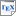[GKKW22] Pascale Gourdeau, Varun Kanade, Marta Kwiatkowska and James Worrell. Sample Complexity Bounds for Robustly Learning Decision Lists against Evasion Attacks. In Proc. 31st International Joint Conference on Artificial Intelligence (IJCAI'22). To appear. July 2022. [pdf] [bib] Downloads:pdf (295 KB)bib Links: [Google] [Google Scholar] [CiteSeer] Abstract. A fundamental problem in adversarial machine learning is to quantify how much training data is needed in the presence of evasion attacks. In this paper we address this issue within the framework of PAC learning, focusing on the class of deci- sion lists. Given that distributional assumptions are essential in the adversarial setting, we work with probability distributions on the input data that satisfy a Lipschitz condition: nearby points have similar probability. Our key results illustrate that the adversary’s budget (that is, the number of bits it can perturb on each input) is a fundamental quantity in determining the sample complexity of robust learning. Our first main result is a sample- complexity lower bound: the class of monotone conjunctions (essentially the simplest non-trivial hypothesis class on the Boolean hypercube) and any superclass has sample complexity at least exponential in the adversary’s budget. Our second main result is a corresponding upper bound: for every fixed k the class of k-decision lists has polynomial sample complexity against a log(n)-bounded adversary. This sheds further light on the question, posed in [Gourdeau et al., 2021], of whether an efficient PAC learning algorithm can always be used as an efficient log(n)-robust learning algorithm under the uniform distribution.